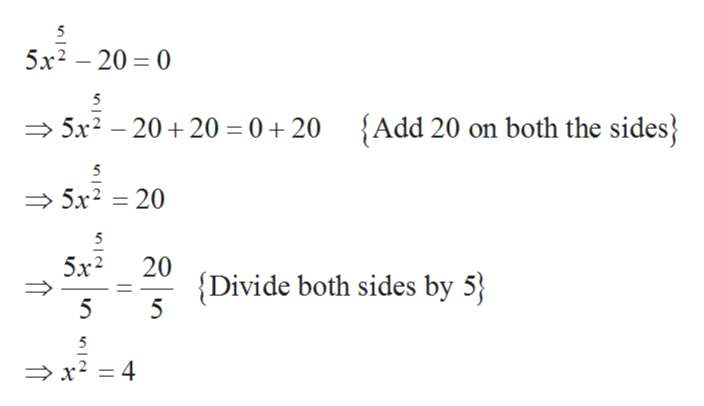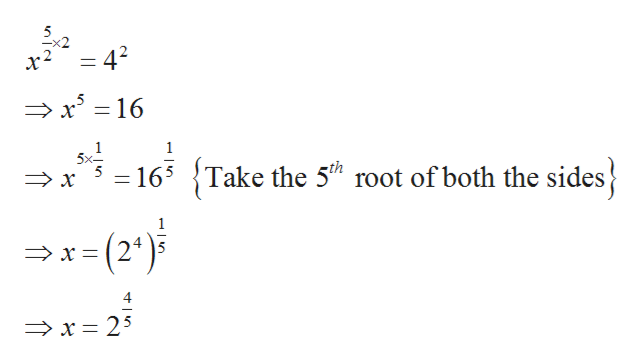5x5/2 -20 = 0

Question

5x5/2 -20 = 0

Step 1

It is required to find the value of the variable x for the equation given below,

Step 2

Solve the equation 5x5/2 – 20 = 0 as,help_outlineImage Transcriptionclose5 5x2 20 0 5 5x2-20+20 0+20 {Add 20 on both the sides 5 5x2 20 5 20 5x2 Divide both sides by 5 5 5 5 x2 = 4 fullscreen
Step 3

Now take the square on both sides of the equa...help_outlineImage Transcriptionclose-x2 = 42 x16 16 5x- Take the 5th root of both the sides x x-(2) 4 x25 fullscreen

Want to see the full answer?

See Solution

Want to see this answer and more?

Our solutions are written by experts, many with advanced degrees, and available 24/7

See Solution
Tagged in

Equations and In-equations